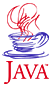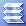Home : Course Map : Chapter 10 : Java : Tech : Bits in JavaIntroduction Vector/Enumeration Hashtable,HashMap    Properties Collections Iterator/ArrayList Generics Preferences API   Demo 1 Concurrency Utils Enumerated Type Arrays Class String Tools   String   StringBuffer   StringBuilder   StringTokenizer   String.split() Calendar,Date,Time   Demo 2   Demo 3 Other Utilities Exercises
 Supplements Performance Benchmarks
 About JavaTech      Codes List      Exercises      Feedback      References      Resources      Tips      Topic Index      Course Guide      What's New

You may occasionally need to access and manipulate data at the bit level. For example, each bit in a set of data might represent the on/off state of a sensor or a pixel in a detector array. Representing the values as bits is much more memory efficient than assigning a full byte to each detector element if an element only holds one of two states.

A more common application of bitwise operations is with colors. We will see in Chapter 11 that the components RGB & alpha (red, green, blue, and the transparency factor) for a color are packed as four byte values into an int value. In image processing and other graphics applications, you can use the bitwise operators to obtain these bytes from a color value, modify the component values, and then pack them back into a int value.

Bitwise Operations

In Chapter 2: Operators we displayed a table of the bitwise operators that act on individual bits in integer types. If the operator involves two operands of different integer types, the wider type will result.

Four of the operators carry out Boolean operations on the bits:

 Compliment: ~x - flips each bit to the opposite value. AND x & y - AND operation between the corresponding bits in x and y. OR x | y - OR operation between the corresponding bits in x and y. XOR x ^ y - XOR operation between the corresponding bits in x and y.

The other three bit operators involved shifting of bits:

 Shift left x << y - shifts x to the left by y bits. The high order bits are lost while zeros fill the right bits. Shift Right - Signed x >> y - shifts x to the right by y bits. The low order bits are lost while the sign bit value (0 for positive numbers, 1 for negative) fills in the left bits. Shift Right - Unsigned x >>> y - shifts x to the right by y bits. The low order bits are lost while zeros fill in the left bits regardless of the sign.

The following code shows how to place color component values into an int variable. We use the hexadecimal format for the literal constants as a compact way to specify byte values.

int [] aRGB = {0x56, 0x78, 0x9A, 0xBC};
int color_val = aRGB;
color_val = color_val | (aRGB << 8);
color_val = color_val | (aRGB << 16);
color_val = color_val | (aRBG << 24);

This results in colorVal holding the value: 56 78 9A BC (separating the byte values for clarity). Similarly, to obtain a particular byte, the code goes like:

int alpha_val = (colorVal >>> 24) & 0xFF;
int red_val   = (colorVal >>> 16) & 0xFF;
int green_val = (colorVal >>>  8) & 0xFF;
int blue_val  =  colorVal & 0xFF;

java.util.BitSet

The BitSet object represents an array of bits whoses size can grow as needed. (In that sense, it is more like a Vector object than a Java array.) The bits represent a set of Boolean values more efficiently by assigning a bit to each true/false value rather than using a whole byte in an array of bytes for an array of boolean primitive types. (JVM implementations for boolean type arrays differ but the Sun JVM uses a byte array.)

The BitSet methods might also be helpful if one is handling data in which individual bits represent information of interest. For example, each bit might represent the state of a relay in a large group of relays. Although internally the JVM might represent the BitSet with an array of long values, there is unfortunately no method in the BitSet class that converts an array of long values into a BitSet or vice versa.

The BitSet class provides methods to access a given bit in the array by an index:

• get (int index)
• set (int index)
• clear (int index)
• flip (int index)

Two BitSet arrays can undergo Boolean operations:

• and (BitSet bitset)
• or (BitSet set)
• xor (BitSet set)
• andNot (BitSet set) - clears all of the bits in the BitSet object when the corresponding bit is set in the specified bitset.

The class includes a number of other methods such as clone() for making copies of a bitset, cardinality() for the number of bits set to one, and nextSetBit(int fromIndex) which returns the index of the next bit set to one at fromIndex or higher.

More Bit Handling

What if a data from another computer platform or an external device comes as int or long values but the bits actually represent float or double values. How would we change an integer type value to the corresponding floating point type? One approach to changing types is discussed in Chapter 9 where we use byte arrays as the destination and source streams. Another approach is to use the methods given below in the Float and Double wrapper classes:

Float

• static int floatToIntBits(float x) - returns an int values whose bits match those of a float according to the IEEE 754 floating-point "single format" bit layout as described in Chapter 2: Tech : Floating Point.

• static int floatToRawIntBits(float value) - same as floatToInBits(float x) except the NaN value can be other than the single IEEE 754 official value (0x7fc00000). As explained in Chapter 2: Tech : Floating Point, a vlaue is NaN if all the bits in the exponent equal 1 and any of the bits in the significand equal 1.

• static float intBitsToFloat(int xbits) - treats the xbits value as though the bits represented a float value and returns it as a float type. That is, this method would convert the output of floatToIntBits(float) above back to a float value.

Double

• static long doubleToLongBits(double x) - returns a long value whose bits match those of the double x value according to the IEEE 754 floating-point bit layout as described in Chapter 2: Tech : Floating Point.

• static long doubleToRawLongBits(double value) - same as doubleToLongBits(double x) except the NaN value can be other than the single IEEE 754 official value (0x7ff8000000000000L). As explained in Chapter 2: Tech : Floating Point, a vlaue is NaN if all the bits in the exponent equal 1 and any of the bits in the significand equal 1.

• static double longBitsToDouble(long bits) - treats the xbits value as though its bits represented a double value and returns it as a double type. That is, this method would convert the output of doubleToLongBits(double) above back to a double value.

The Integer wrapper also offers some bit handling methods:

• static String toBinaryString(int i) - converts the value i to a string with 0 and 1 characters but no leading zeros.
• static int parseInt(String s, int radix) - conversely, if s is a string representing a binary value, such as "110103", and radix is set to 2, then the method will return an int value equal to the binary value.

Similarly, the Long wrapper has a two methods of the same names for converting long values to binary strings and strings that represent binary values to long values.

Note that the BigInteger class contains bitwise methods to test bits, shift bits, clear a particular bit, and so forth. However, remember that any change of a bit results in a new BigInteger object since instances of this class are immutable

References & Web Resources

Latest update: Nov. 19, 2004

 Tech ArbitaryPrecision    BigInteger    BigDecimal Bit Handling Exercises
 Physics Data Gen&Analysis   Demo 1   Demo 2 ExercisesPart I Part II Part III Java Core 1  2  3  4  5  6  7  8  9  10  11  12 13 14 15 16 17 18 19 20 21 22 23 24 Supplements 1  2  3  4  5  6  7  8  9  10  11  12 Tech 1  2  3  4  5  6  7  8  9  10  11  12 Physics 1  2  3  4  5  6  7  8  9  10  11  12

Java is a trademark of Sun Microsystems, Inc.